# Creating Heatmap Using Python Seaborn

In our previous blog, we talked about Data Visualization in Python using Bokeh. Now, let’s take our series on Python data visualization forward, and cover another cool data visualization Python package. In this post, we will use the Seaborn Python package to create Heatmaps which can be used for various purposes, including by traders for tracking markets.## Seaborn for Python Data Visualization

Seaborn Python is a data visualization library based on Matplotlib. It provides a high-level interface for drawing attractive statistical graphics. Because seaborn python is built on top of Matplotlib, the graphics can be further tweaked using Matplotlib tools and rendered with any of the Matplotlib backends to generate publication-quality figures. 

Types of plots that can be created using seaborn python include:

• Distribution plots
• Regression plots
• Categorical plots
• Matrix plots
• Time series plots

The plotting functions operate on Python data frames and arrays containing a whole dataset and internally perform the necessary aggregation and statistical model-fitting to produce informative plots.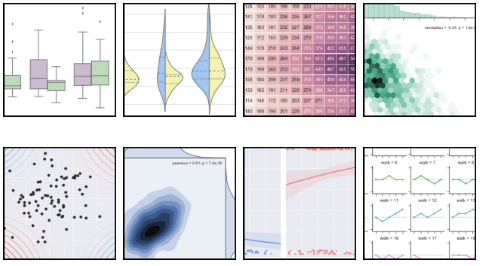Source: seaborn.pydata.org

## What is a heatmap?

A heatmap is a two-dimensional graphical representation of data where the individual values that are contained in a matrix are represented as colors. The seaborn python package allows the creation of annotated heatmaps which can be tweaked using Matplotlib tools as per the creator’s requirement.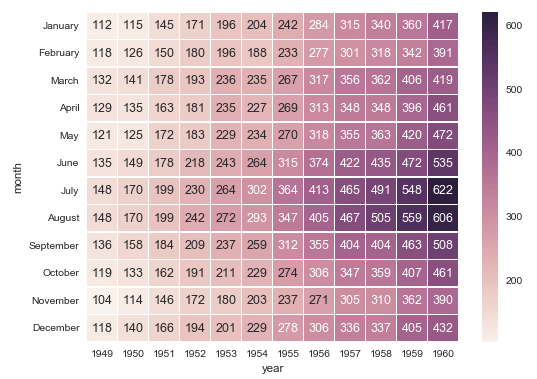Annotated Heatmap

## Python Heatmap Code

We will create a seaborn heatmap for a group of 30 Pharmaceutical Company stocks listed on the National Stock Exchange of India Ltd (NSE). The seaborn heatmap will display the stock symbols and its respective single-day percentage price change.

We collate the required market data on Pharma stocks and construct a comma-separated value (CSV) file comprising of the stock symbols and their respective percentage price change in the first two columns of the CSV file.

Since we have 30 Pharma companies in our list, we will create a heatmap matrix of 6 rows and 5 columns. Further, we want our seaborn heatmap to display the percentage price change for the stocks in a descending order. To that effect, we arrange the stocks in a descending order in the CSV file and add two more columns which indicate the position of each stock on X & Y axis of our heatmap.

## Import the required Python packages

We import the following Python packages: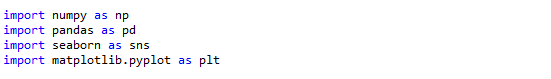We read the dataset using the read_csv function from pandas and visualize the first ten rows using the print statement.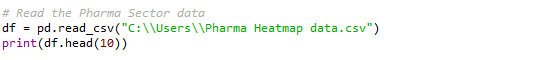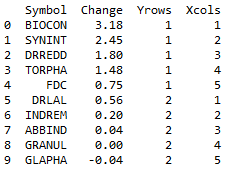### Create a Python Numpy array

Since we want to construct a 6 x 5 matrix, we create an n-dimensional array of the same shape for “Symbol” and the “Change” columns.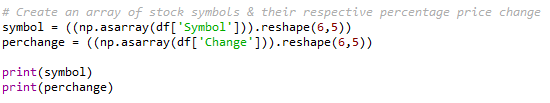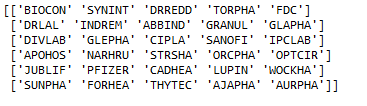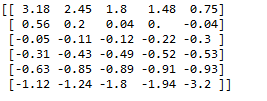### Create a Pivot in Python

The pivot function is used to create a new derived table from the given data frame object “df”. The function takes three arguments; index, columns, and values. The cell values of the new table are taken from column given as the values parameter, which in our case is the “Change” column.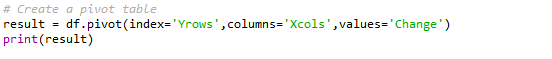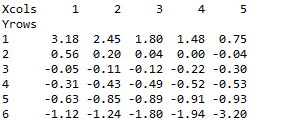### Create an Array to Annotate the Heatmap

In this step, we create an array which will be used to annotate the seaborn heatmap. We call the flatten method on the “symbol” and “percentage” arrays to flatten a Python list of lists in one line. The zip function which returns an iterator zips a list in Python. We run a Python For loop and by using the format function; we format the stock symbol and the percentage price change value as per our requirement.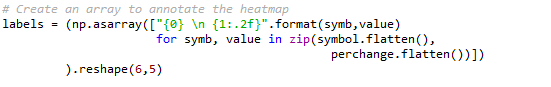### Create the Matplotlib figure and define the plot

We create an empty Matplotlib plot and define the figure size. We also add the title to the plot and set the title’s font size, and its distance from the plot using the set_position method.

We wish to display only the stock symbols and their respective single-day percentage price change. Hence, we hide the ticks for the X & Y axis, and also remove both the axes from the heatmap plot.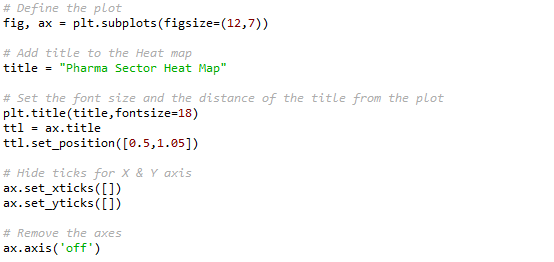### Create the Heatmap

In the final step, we create the heatmap using the heatmap function from the seaborn python package. The heatmap function takes the following arguments:

data – 2D dataset that can be coerced into an ndarray. If a Pandas DataFrame is provided, the index/column information will be used to label the columns and rows.

annot – an array of same shape as data which is used to annotate the heatmap.

cmap – a matplotlib colormap name or object. This maps the data values to the color space.

fmt – string formatting code to use when adding annotations.

linewidths – sets the width of the lines that will divide each cell.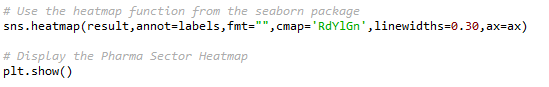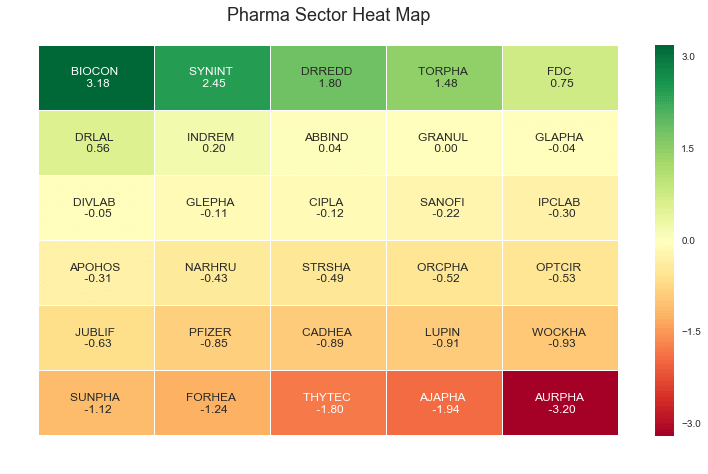Here’s our final output of the seaborn python heatmap for the chosen group of pharmaceutical companies. Looks pretty neat and clean, doesn’t it? A quick glance at this heatmap and one can easily make out how the market is faring for the period.

Readers can download the entire seaborn Python code plus the excel file using the download button provided below and create their own custom heatmaps. A little tweak in the Python code and you can create seaborn Python heatmaps of any size, for any market index, or for any period using this Python code. The seaborn heatmap can be used in live markets by connecting the real-time data feed to the excel file that is read in the Python code.

## To Conclude

As illustrated by the heatmap example above, seaborn python is easy to use and one can tweak the seaborn plots to one’s requirement. You can refer to the documentation of seaborn for creating other impressive charts that you can put to use for analyzing the markets.

## Next Step

Learn the step-by-step process to understand and compute VaR in Excel and Python using Historical Method and Variance-Covariance approach in our post Calculating Value At Risk In Excel & Python. You can also download the excel and Python codes to calculate the VaR for yourself.